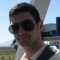Home » Core Java » lang » Java hashCode method ExampleAldo is a student of Computer Engineering and a programming addict. He spares his free time coding, whether mobile, web, or desktop programming. He is also one of the co-founders of Things Lab.

# Java hashCode method Example

In this example we will discuss about `hashCode()` method. This is one of the methods that all objects have, since it is defined in the `Object` class, which is the base for all Java classes.

This method’s functionality is to digest the properties of an object into a single, 32-bit integer value. The `hashCode()` method should return a unique value for every object, and two objects must not have the same integer hash value, unless they are equal as the `equals()` method says.

The programmer is not obligated to override this method, since the base method defined in the `Object` class works perfectly (i.e. returns different values for different objects).

## Example of hashCode() usage

With the following example, I will show that the hash value returned by `hashCode()` is the same for objects that `equals()` returns true; and it differs when the `equals()` returns false. Create a class called `BasicHashCodeExample` with the following source code:

```package com.javacodegeeks.examples;

public class BasicHashCodeExample {

public static void main(String[] args) {

String name1 = "John";
String name2 = "Jane";
String name3 = "John";

System.out.println("name1.equals(name2): "+name1.equals(name2));
System.out.println("name1.hashCode() = "+name1.hashCode());
System.out.println("name2.hashCode() = "+name2.hashCode());

System.out.println("name1.equals(name3): "+name1.equals(name3));
System.out.println("name1.hashCode() = "+name1.hashCode());
System.out.println("name3.hashCode() = "+name3.hashCode());
}

}
```

When executing this, the output will be:

```name1.equals(name2): false
name1.hashCode() = 2314539
name2.hashCode() = 2301262
name1.equals(name3): true
name1.hashCode() = 2314539
name3.hashCode() = 2314539
```

You can notice here that `name1` and `name3` have the same hash value, while `name1` and `name2` don’t!

## hashCode() in action

Normally, hash values are used in hash tables or other data structures that use hash values for indexing individual objects in the collection. Let’s take a collection example, like a list of people names. You could store them in a simple `String` array, or in an `ArrayList`, or better yet in a `TreeSet`. To search a name in the list, you would use a searching algorithm, like linear search, or binary search in the case of the `TreeSet`, but they would take too much time if the list of names is big. In terms of big-O, linear search and binary search have complexities of O(n) and O(log(n)), respectively. But, if you decide to store the list of people names in a hash table for example, the complexity of searching would be O(1)! And that’s really faster!

To show how to search in a `HashMap`, an implementation of a hash table based on the `Map` interface, check the following example:

```package com.javacodegeeks.examples;

import java.util.HashMap;
import java.util.Map;
import java.util.Random;

public class HashMapExample {

public static void main(String[] args) {
Map hashTable = new HashMap();
Random rnd = new Random();

String baseName = "Base_Name";
for (int i=0;i<100;i++) {
String tmpName = baseName+i;
int rndAge = rnd.nextInt(70);

Person p = new Person(tmpName, rndAge);

hashTable.put(tmpName.hashCode(), p);
}

//now let's find the person with name Base_Name75

String name = "Base_Name75";
Person person = hashTable.get(name.hashCode());
System.out.println(person.getName());
}

static class Person {
private String name;
private int age;

public Person(String name, int age) {
this.name = name;
this.age = age;
}

public String getName() {
return this.name;
}

public int getAge() {
return this.age;
}
}
}
```

Initially, we create a `HashMap` instance to store instances of a `Person` class using an integer as key. On the for loop, we create an instance of `Person` class with a random age and a name that is “Base_Name” plus an index. Then we store this instance into the hash table, using the hash value of the name as key.

Next, we use the hash value of the name we want to get the person instance from the hash table.

The output will be like this:

```Base_Name75
```

The general contract of hashCode is:

• Whenever it is invoked on the same object more than once during an execution of a Java application, the hashCode method must consistently return the same integer, provided no information used in equals comparisons on the object is modified. This integer need not remain consistent from one execution of an application to another execution of the same application.
• If two objects are equal according to the equals(Object) method, then calling the hashCode method on each of the two objects must produce the same integer result.
• It is not required that if two objects are unequal according to the equals() method, then calling the hashCode method on each of the two objects must produce distinct integer results. However, the programmer should be aware that producing distinct integer results for unequal objects may improve the performance of hash tables.
• As much as is reasonably practical, the hashCode method defined by class Object does return distinct integers for distinct objects. (This is typically implemented by converting the internal address of the object into an integer, but this implementation technique is not required by the JavaTM programming language.)

You can download the full source code of this example here : HashCodeExample

# Do you want to know how to develop your skillset to become a Java Rockstar?

## Subscribe to our newsletter to start Rocking right now!

### and many more ....